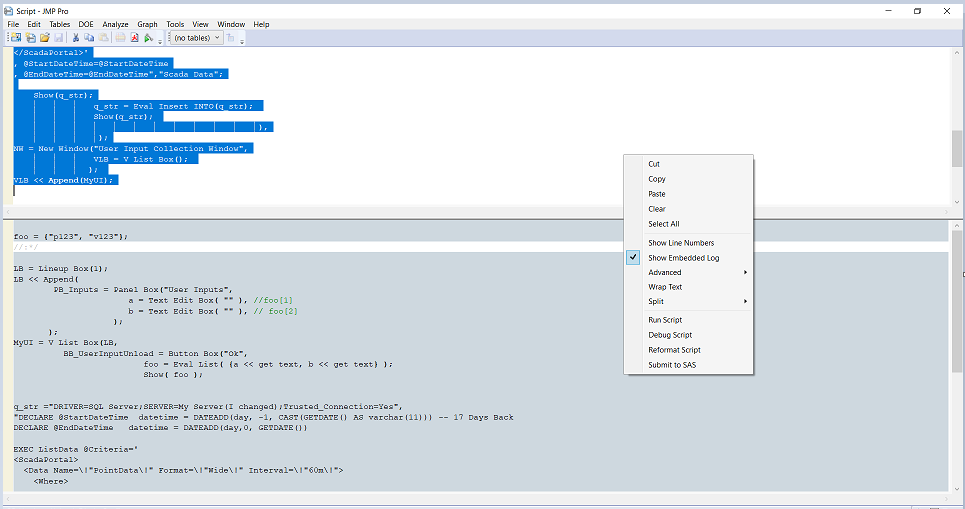Choose Language Hide Translation Bar
Highlighted

## Re: A variable value from user - sql query

What I posted is a log - see belowThe log -

``````foo = {"p123", "v123"};
//:*/

LB = Lineup Box(1);
LB << Append(
PB_Inputs = Panel Box("User Inputs",
a = Text Edit Box( "" ), //foo
b = Text Edit Box( "" ), // foo
);
);
MyUI = V List Box(LB,
foo = Eval List( {a << get text, b << get text} );
Show( foo );

q_str ="DRIVER=SQL Server;SERVER=My Server(I changed);Trusted_Connection=Yes",
"DECLARE @StartDateTime  datetime = DATEADD(day, -1, CAST(GETDATE() AS varchar(11))) -- 17 Days Back
DECLARE @EndDateTime   datetime = DATEADD(day,0, GETDATE())

EXEC ListData @Criteria='
<Data Name=\!"PointData\!" Format=\!"Wide\!" Interval=\!"60m\!">
<Where>

<Column Name=\!"Point\!" Project=\!"^foo^\!" Value=\!"^foo^\!"/>

</Where>
</Data>
, @StartDateTime=@StartDateTime

Show(q_str);
q_str = Eval Insert INTO(q_str);
Show(q_str);
),
);
NW = New Window("User Input Collection Window",
VLB = V List Box();
);
VLB << Append(MyUI);

/*:``````

Are you sure that i "defining strings with separators outside the quotes" ?  Plese, can you mark the separators and tell me what do you mean.

* The issue is that when I run the script and insert values to  `Panel Box`   and than i press to   `Button Box("Ok"..)`  ,   I dont recived any data.

Thx.

Highlighted

## Re: A variable value from user - sql query

Please post your log. This script is not running a query, it is just writing the result of the query command to the log.

Which version of JMP are you using?  JMP has a Query Builder.  You could create that query to prompt for the two fields you need. Then you can save the script, or the query.  There are multiple points of failure for a query,

• Incorrect query syntax, note that case can be important.  So if a user is typing in harry, but the db field is HARRY, then the result will be empty.  Also SQL quires quotes around a value with spaces or special characters. So first, get a script to work without the prompt, that is hardcode the values of the prompt and run the portion of code   dtScadaData =  Open Database(etc.)
•  Once the hardcoded script works, then try the prompt typing in exactly what was hardcoded above.  Also print out  the string and compare.mple,
• The query login can be a point of failure
• Your connection string to the db driver may not be the same as another users.  For example, I set up the driver for Excel to be named Excel Files, someone else might have a different name.
• Other user privileges might be different than yours, they might not have access to the files/tables.
• I am sure there are more,

I see that @uday_guntupalli just responded. So I will stope now.

Highlighted

## Re: A variable value from user - sql query

@Botzal, please search for Open Database() in the JMP Scripting Index >> Functions. This function has 2 required and 2 option arguments:

1. the connect string
2. the query string
3.  <invisible | private>  property for the output table, you likely do not want this
4. <output table name>

You have been using one string for the first two arguments.  It is difficult to debug and see this when we do not have test files to use. Remove the comment lines for dt_ScadaData to test if it runs. Remove the comment lines for NW if you want the UI to automatically close. You might want it to remain open so you can pull several tables. If that is what you want, then Eval Insert Into(qstr),  replaces the place holder variables foo and foo.   So just in case you intend to use the UI more than once, I created a variable cq_str which is assigned the variable string. So this should work.  I typically do not use Eval Insert Into() but instead something like cq_str = Eval Insert(q_str);

``````LB = Lineup Box(1);
LB << Append(
PB_Inputs = Panel Box("User Inputs",
a = Text Edit Box( "" ), //foo
b = Text Edit Box( "" ), // foo
);
);
q_connect = "DRIVER=SQL Server;SERVER=MyServer;Trusted_Connection=Yes";
q_str = "DECLARE @StartDateTime  datetime = DATEADD(day, -2, CAST(GETDATE() AS varchar(11)))
DECLARE @EndDateTime   datetime = DATEADD(day,0, GETDATE())
EXEC ListData @Criteria=
<Data Name=\!"PointData\!" Format=\!"Wide\!" Interval=\!"60m\!">
<Where>
<Column Name=\!"Point\!" Project=\!"^foo^\!" Value=\!"^foo^\!"/>
</Where>
</Data>
, @StartDateTime=@StartDateTime
, @EndDateTime=@EndDateTime

MyUI = V List Box(LB,
foo = Eval List( {a << get text, b << get text} );
Show( foo );
cq_str = q_str;
Show(cq_str);
Eval Insert Into(cq_str);
Show(cq_str);
//NW << Close Window(); //this will close the UI don't use if you want to keep ist open
) //end ButtonBox
);  //end VListBox
NW = New Window("User Input Collection Window",
VLB = V List Box();
);
VLB << Append(MyUI);``````

Highlighted

## Re: A variable value from user - sql query

Hi All,

@gzmorgan0 , Thank you so much for your solution, that works good!

@uday_guntupalli @stan_koprowski @Jeff_Perkinson ,Thank you all for your support!

Article Labels

There are no labels assigned to this post.